# Colleges with the lowest SAT scores in Missouri

Top 10 colleges in Missouri with the lowest SAT scores
Looking for the colleges with the lowest SAT scores in Missouri? Well you're in luck! We've compiled a national college database and have created a list of the top 10 universities with the lowest SAT scores in Missouri below. If you are not a good test taker or worried about your test scores, this list is for you. These are the schools whose applicants had the lowest average SAT scores in Missouri, which means that you can get into these colleges with a lower SAT score. We also include each college's ACT scores and acceptance rate so that you can see where you would have the easiest time getting in. Read on to find out more.

## Harris-Stowe State University SAT scores

The average SAT score for Harris-Stowe State University is 880.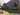The average SAT score of 880 breaks down into:

• SAT math: 430

The average ACT score for Harris-Stowe State University is 18 and their acceptance rate is 55.7%.

## Avila University SAT scores

The average SAT score for Avila University is 930.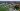The average SAT score of 930 breaks down into:

• SAT math: 490

The average ACT score for Avila University is 22 and their acceptance rate is 41.1%.

## Central Methodist University SAT scores

The average SAT score for Central Methodist University is 1030.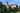The average SAT score of 1030 breaks down into:

• SAT math: 520

The average ACT score for Central Methodist University is 23 and their acceptance rate is 62.6%.

## Culver-Stockton College SAT scores

The average SAT score for Culver-Stockton College is 1050.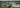The average SAT score of 1050 breaks down into:

• SAT math: 520

The average ACT score for Culver-Stockton College is 21 and their acceptance rate is 46.4%.

## Hannibal-LaGrange University SAT scores

The average SAT score for Hannibal-LaGrange University is 1070.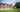The average SAT score of 1070 breaks down into:

• SAT math: 510

The average ACT score for Hannibal-LaGrange University is 22 and their acceptance rate is 56%.

## Evangel University SAT scores

The average SAT score for Evangel University is 1080.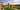The average SAT score of 1080 breaks down into:

• SAT math: 520

The average ACT score for Evangel University is 22 and their acceptance rate is 61%.

## Lindenwood University SAT scores

The average SAT score for Lindenwood University is 1080.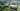The average SAT score of 1080 breaks down into:

• SAT math: 540

The average ACT score for Lindenwood University is 22 and their acceptance rate is 88.1%.

## Missouri Valley College SAT scores

The average SAT score for Missouri Valley College is 1080.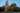The average SAT score of 1080 breaks down into:

• SAT math: 580

The average ACT score for Missouri Valley College is 21 and their acceptance rate is 53.7%.

## Southwest Baptist University SAT scores

The average SAT score for Southwest Baptist University is 1104.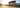The average SAT score of 1104 breaks down into:

• SAT math: 550

The average ACT score for Southwest Baptist University is 22 and their acceptance rate is 70.6%.

## Missouri Southern State University SAT scores

The average SAT score for Missouri Southern State University is 1110.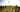The average SAT score of 1110 breaks down into:

• SAT math: 555